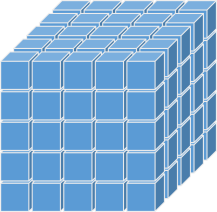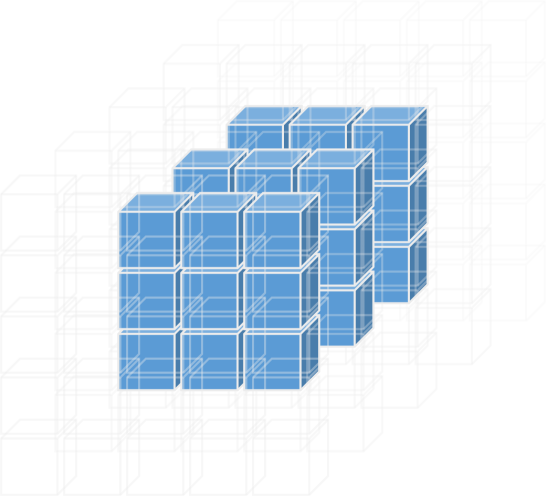# Another Painted Cube Puzzle

I've written about the classic Painted Cube Puzzle in the past. In the earlier example all sides of the cube were painted. In this new variant, an unknown number of sides are painted, and also, the size of the cube is unknown. Satisfyingly, however, there is enough information to solve it.## Puzzle

You have a collection of unit cubes which you connect together to make a complete larger cube. Then, an unkown number of the sides of this larger cube are sprayed with red paint. After the paint has dried, the larger cube is deconstucted back into a pile of unit cubes. After deconstruction you count, and find that exactly 45 of the small cubes have no paint on them. How many of the faces of where painted, and how big was the larger cube?## Breaking it down

First, let's break the puzzle down (see what I did there?)
Let's define n to be the length of the side of the larger cube.
This means there are n3 smaller cubes in the larger cube.
There is no way that any of the 'inner' cubes can get paint on them (they have no external sides). If the larger cube has dimension n, then there are (n-2)3 inner cubes.None of the inner cubes can get paint. Even if every one of the outer gets paint (meaning that the only unpaintned cubes are the inners), we know that there are 45 unpainted cubes. Therefore (n-2)3 ≤ 45.
From this, (n-2) ≤ 3 and gives the result: n ≤ 5.
The larger cube cannot have a length greater than five, otherwise we'd have found more unpainted cubes.
We can also define a lower bound. We have 45 unpainted cubes, so we known that there must be at least this number of cubes in total. This gives another inequality of n3 ≥ 45. Because the number of cubes needs to be an integer, then n ≥ 4.
From these two inequalities we know there are only possible answers n = 4 or n = 5.
The cube is either four or five units a side.

## n = 4

Let's examine the case of n = 4 first.If the larger cube is four units, this means there are eight inner cubes.
The number of outer cubes is 43 – 8 = 56.
There are 56 external cubes.
We know that 45 cubes are unpainted, and 8 of these are internal. This means that 37 of the extenal cubes also have to have no paint.
The complement of this is that 19 of the external cubes will need to have some paint on them.
We have to be careful counting the painted cubes because we don't want to double count any cube (if two adjacent sides are painted, the cubes on the common edge are painted twice, but we only need to count them once. Similarly on the corners).
Each face of the larger cube contains 16 smaller cubes. We need 19 cubes to be painted in total, so if just one face were painted, we're short. Therefore at least two faces would have had to have been painted. If we painted two opposite faces, we'd have 32 smaller cubes with paint on them; too many! If we painted two adjacent faces, we'd have 28 painted cubes (16 from the first edge and 4×3 from the adjacent edge; the four common edge cubes having been de-duped). This is too many! If we painted more faces it only gets worse. There is no way the larger cube can have edge 4.
There is no way the larger cube can have edge four

## n = 5

If there is a solution, then it has to be n = 5.Repeating the same calculations:
There are (3×3×3) = 27 internal cubes.
There are (5×5×5) = 125 cubes in total.
There are 125-27 = 98 external cubes.
Of these external cubes 45-27 = 18 need to be unpainted.
Therefore, we need to see if we can get 80 painted cubes.

## How many faces were painted?

If all six external faces were painted, we'd only have the inner cubes unpainted, and this is too few.
If five of the external faces were painted, this leaves one face clean. De-duping the common edges results in just (3×3) unpainted cubes in the middle of that face. When added to the 27 internal cubes, this is not enough.
If four external faces were painted this leaves two clean faces. There are two possible ways this can occur. The clean faces can be on opposite sides of the cube, or they could be adjacent to each other (without loss of generality). If the clean faces are adjacent, there are 21 outer clean cubes; 21+27 = 48, which is too many. If the clean faces are opposite, this gives (2×9)+27 = 45. This is the answer we are looking for!
(We can confirm that if we painted three or less, then there is no way ot make it. We need at least 80 cubes to be painted and, even ignoring the common edges, there's, at most, 25 a side, so we can't have three or fewer).
Here it is shown as a cube mesh. The painted faces are show in red. The common edge cubes are orange (they are not painted but are counted as painted). Faces that show cubes that are counted as painted have a cross on them.There cube is size (5×5×5), and four sides are painted.